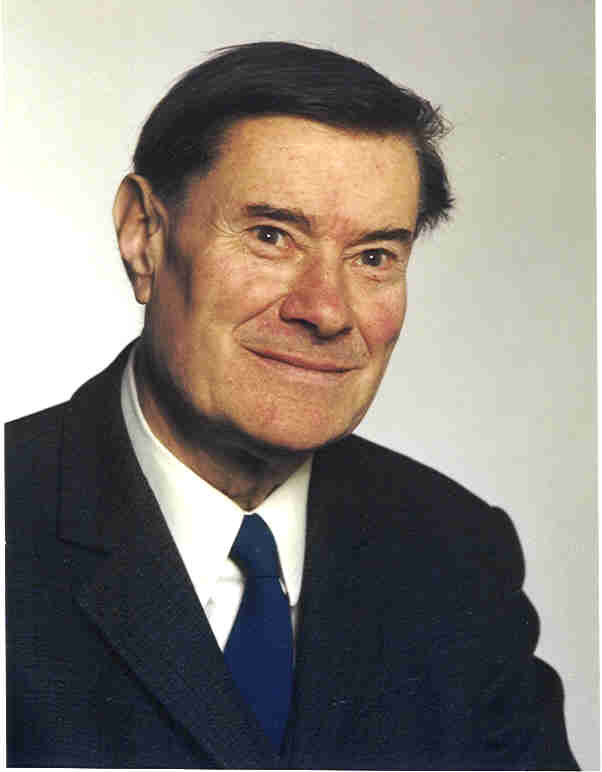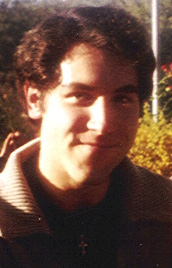# The existence of half-units e = (x + y * d1/2)/2

## in real quadratic number fields K = Q(d1/2).## The inspiration.

During the start and the completion of the half-unit project,
I was definitely feeling much internal inspiration and motivation
by the spirit of my honourable academic teacher
Alexander Aigner (May 18, 1909 - June 7, 1988).
Here I express my respect and gratitude to him
for helping me to succeed in this enterprise.*

## Preliminaries.

It is well known that the maximal order O1 of a quadratic number field K = Q(d1/2)
contains half-integers of the shape (x + y*d1/2)/2 with coprime rational integers x,y in Z,
if and only if the discriminant d of K is congruent 1 modulo 4, d = 4*n + 1 (n in Z).

In the other cases, d = 0(mod 4) with d/4 = 2(mod 4) or d/4 = 3(mod 4),
the ring O1 of algebraic integers of K consists only of elements of the shape x + y*d1/2 with x,y in Z.

The proof does not use more than High School Algebra.
*

## The start in 1988.

However, it is not well known which conditions must be satisfied in the case d = 1(mod 4) of a real quadratic field K
in order that the fundamental unit e of K is a half-integer, i. e., a so called half-unit [1, � 49, p. 174, ff.].

A first general restriction is provided by the prime residue class group U(O1/F) of the conductor F = 2*O1
of the suborder O2 = Z*1 + Z*d1/2 in the maximal order O1 = Z*1 + Z*(1 + d1/2)/2,
with unit groups U2 and U1, respectively ([2, p. 42, Proposition 4.1]):
for d = 1(mod 8), 2 splits in K, U(O1/F) = C1, and (U1:U2) = 1, whereas
for d = 5(mod 8), 2 remains inert in K, U(O1/F) = C3, and (U1:U2) = 1 or 3.

 Thus, there are certainly no half-units, if d = 1(mod 8), but there may exist half-units, i. e., (U1:U2) = 3, if d = 5(mod 8).
*
A necessary and sufficient condition for the existence of half-units in the case d = 5(mod 8)
is provided by the formula for the ring class number modulo 2 of K:
[F2|K] = 3 * [F1|K] / (U1:U2),     which yields the following

 Theorem 1. (for the proof see [2, p. 50, Theorem 4.4]) The 3-ring class field F2 modulo 2 of K is a cyclic cubic extension of the Hilbert 3-class field F1 of K if and only if there are no half-units in K: [F2|F1] = 3     < == >     (U1:U2) = 1

A drawback of this theorem is that testing the class field theoretic condition
is even harder than directly testing the index of the unit groups by means of continued fractions.

However, for real quadratic fields with class number coprime to 3, i. e., with 3-class rank zero,
the above Theorem 1 changes to the following convenient criterion

 Theorem 2. (for the proof see [2, p. 50, Theorem 4.4]) There are no half-units in K if and only if there exists an absolute (non-Galois) cubic extension L|Q with discriminant 4*d.
*

## The completion in 2008.

Chapter 4 of the tract  had a considerable influence on the development of my theory of
discriminantal multiplicities of p-ring class fields over quadratic fields in my paper .
This theory reached its highlights by the introduction of the concepts of p-ring spaces and p-defects
in my presentation Wiener Kongress 2001 .

Now it turned out that the impact of p-ring spaces Vp(f) mod f is not limited to counting dihedral fields of degree 2p
but can also be specialized to the problem of the existence of half-units by taking p = 3 and f = 2.
Since f = 2 is a prime conductor, the 3-ring space V3(2) mod 2 is
at most of codimension 1 in the F3-vector space V3 of non-trivial generators of ideal cubes,
i. e., the 3-defect of 2 is bounded by 0 <= d3(2) <= 1.

The following Theorem 3 extends Theorem 1 by distinguishing three cases
in terms of 3-deficient elements mod 2 of V3.

 Theorem 3. (for the proof see ) Let K be a real quadratic field with discriminant d = 5(mod 8) and 3-class rank r. Denote the 3-ring class field modulo 2 of K by F2 and the Hilbert 3-class field of K by F1. Further let e be the fundamental unit of K and a1,...,ar the other generating ideal cubes of the (r+1)-dimensional F3-vector space V3 = < e,a1,...,ar >. 1. (Case with a 3-deficient unit mod 2) There exist half-units in K if and only if F2 coincides with F1. (Then e in O1 but a1,...,ar in O2.) 2. Half-units do not exist in K if and only if a) (Case with a 3-deficient non-unit mod 2) either F2 is a non-split extension of F1 over K (Then e in O2 and exactly one of a1,...,ar in O1 and there exist 3r-1 cyclic nonic extensions N~|K with conductor 2.) b) (Case without 3-deficient ideal cubes mod 2) or F2 is a split extension of F1 over K. (Then e,a1,...,ar in O2 and there exist 3r non-isomorphic cubic extensions L|Q with discriminant 4*d.)
*

Now we illustrate Theorem 3 by a table which shows the minimal discriminants of all known configurations,
ordered by increasing 3-class rank of the real quadratic fields K.
For 3-class rank zero, the non-split extension case cannot occur.
The letter w denotes the generating surd d1/2 of K.

3-class
rank
Gal(F1|K)DiscriminantFundamental unitGal(F2|K)Generators of ideal cubes3-ring space
mod 2
3-defect
of 2
015e = (1 + w)/2 in O11--O1
0137e = 6 + w in O2(3)--[e]0
1(3)229e = (15 + w)/2 in O1(3)a = 2391 + 158*w in O2[a]1
1(3)1765e = 42 + w in O2(9)a = (303 + 7*w)/2 in O1[e]1
1(3)7053e = 4703 + 56*w in O2(3,3)a = 86 + w in O2[e,a]0
2(3,3)130397e = (6861 + 19*w)/2 in O1(3,3)a = 8521509330 + 23598409*w in O2
b = 42513400012 + 117731327*w in O2
[a,b]1
2(3,3)62501e = 250 + w in O2(9,3)a = 8110567 + 32442*w in O2
b = (251 + w)/2 in O1
[e,a]1
2(3,3)4562765e = 123715750188465719
+ 57917651345508*w in O2
(3,3,3)a = 7259 + 10*w in O2
b = 576737 + 270*w in O2
[e,a,b]0
3(9,3,3)925219837e in O2(9,3,3,3)a in O2, b in O2, c in O2[e,a,b,c]0

Remark: In the cases with 3-defect d3(2) = 1,
the first generating ideal cube a = (x + y*w)/2 encountered in
solving the corresponding Diophantine norm form equation x2 - d*y2 = 4*q3
is not always an element of O2 but frequently of O1.
Then e*a, e2*a, b*a or b2*a is in general an element of O2.

Examples:
For d = 229, we have a = (27 + w)/2, e*a = (317 + 21*w)/2, e2*a = 2391 + 158*w.
For d = 130397, we have a = (363 + w)/2, e*a = (2484043 + 6879*w)/2, e2*a = 8521509330 + 23598409*w
and b = (1807 + 5*w)/2, e*b = (12392771 + 34319*w)/2, e2*b = 42513400012 + 117731327*w.
For d = 62501, we have a = (267 + w)/2, b*a = (64759 + 259*w)/2, b2*a = 8110567 + 32442*w.

*
Finally we conclude with Class Field Diagrams for the minimal discriminants of all known configurations.

 K = Q(51/2) = F1 = F2 | Q
 N1 = F2 | K = Q(371/2) = F1 | Q
 N^1 = F1 = F2 | K = Q(2291/2) | Q
 N~1 = F2 | N^1 = F1 | K = Q(17651/2) | Q
 F2 / / \ \ N^1 = F1 N1 N2 N3 \ \ / / K = Q(70531/2) | Q
 F1 = F2 / / \ \ N^1 N^2 N^3 N^4 \ \ / / K = Q(1303971/2) | Q
 F2 / / \ \ F1 N~1 N~2 N~3 / / \ \ \ / / N^1 N^2 N^3 N^4 \ \ / / K = Q(625011/2) | Q
 F2 / | \ F1 ... \ \ \ \ / / | | | | | | | N^1 N^2 N^3 N^4 N1 N2 N3 N4 N5 N6 N7 N8 N9 \ \ \ \ \ \ | / / / / / / K = Q(45627651/2) | Q

Final Note: Some of these minimal discriminants occurred in other context already.
First, d = 62501 as the smallest example of family principal factorization type (Alpha1,Alpha1,Alpha1,Alpha1) .
Second, d = 4*4562765 = 18251060 and d = 4*925219837 = 3700879348 as the minimal discriminants with multiplicity 9 and 27 .

 Bibliographic References:  Alexander Aigner, Zahlentheorie, Walter de Gruyter, Berlin, 1975.  Daniel C. Mayer, Lattice minima and units in real quadratic number fields, Publicationes Mathematicae Debrecen 39 (1991), 19-86.  Daniel C. Mayer, Multiplicities of dihedral discriminants, Mathematics of Computation 58 (1992), no. 198, 831-847, and Supplements section S55-S58.  Daniel C. Mayer, Ring spaces for counting dihedral fields, Univ. of Manitoba, Dept. of Computer Science, Latest update, 2008.

 * Web master's e-mail address: contact@algebra.at *